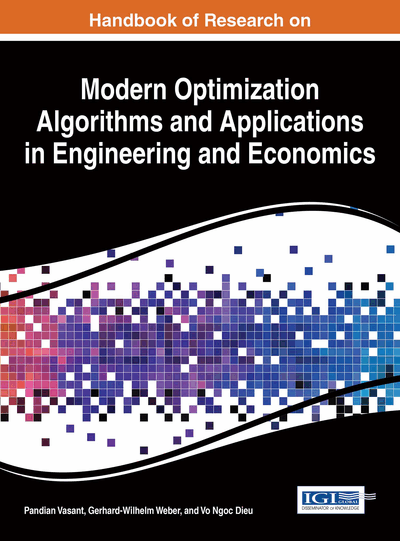# Single Batch-Processing Machine Scheduling Problem with Fuzzy Due-Dates: Mathematical Model and Metaheuristic Approaches

DOI: 10.4018/978-1-4666-9644-0.ch028

## Abstract

This paper focuses on a problem of minimizing total weighted tardiness of jobs in a real-world single batch-processing machine (SBPM) scheduling in existence of fuzzy due date. In this paper, first a fuzzy mixed integer linear programming model is developed. Then, due to the complexity of the problem, which is NP-hard, we design two hybrid metaheuristics called GA-VNS and VNS-SA applying the advantages of genetic algorithm (GA), variable neighborhood search (VNS) and simulated annealing (SA) frameworks. Besides, we propose three fuzzy earliest due date heuristics to solve the given problem. Through computational experiments with several random test problems, a robust calibration is applied on the parameters. Finally, computational results on different-scale test problems are presented to compare the proposed algorithms.
Chapter Preview
Top

## 1. Introduction

A batch processing machine (BPM) is a special variant of a scheduling problem, in which several jobs can be simultaneously processed in such a way that all the jobs in a batch start and complete their processing at the same time. The main advantage is to reduce setups and/or facilitation of material handling. The problem of BPM scheduling is often encountered in real industries. The industrial application of these machines can be found in semiconductor burn-in operations, environmental stress-screening (ESS) chambers, chemical, food and mineral processing, pharmaceutical and construction materials industries, etc.

The BPM scheduling problem is important because the scheduling of batching operations has a significant economic impact. It is mainly motivated by an industrial application, namely, the burn-in operation found in the final testing phase in semiconductor manufacturing (Uzsoy et al., 1992; Uzsoy et al., 1994). In the semiconductor manufacturing, the jobs have different processing times and sizes that are both required by the customers. The jobs are grouped in batches where a batch means a subset of jobs. The BPM can process a batch of jobs as long as the sum of all the job sizes in the batch does not violate the capacity of the machine. The processing time of a batch is equal to the longest processing time of all the jobs in that batch.

Ikura and Gimple (1986) were the first researcher who studied the BPM problem and Lee et al. (1992) first presented a detailed description for burn-in operation. As reported in the studies, the exact algorithms have a slow convergence rate and they can solve only small instances to optimality.

As this study addresses SBPM with fuzzy due dates using metaheuristics, the review on SBPM scheduling under a fuzzy environment and the application of metaheuristics to these problems is carried out. For an extensive review on BPM scheduling problems, we refer to Potts and Kovalyov (2000) and Mathirajan and Sivakumar (2006).

In BPM scheduling problems, Wang and Uzsoy (2002) firstly proposed a metaheuristic algorithm. Considering dynamic job arrivals, they combined a dynamic programming algorithm with a random key genetic algorithm (GA) to minimize the maximum lateness. Melouk et al. (2004) used a simulated annealing (SA) to minimize the makespan. Koh et al. (2005) proposed a random key representation-based GA for the problems of minimizing the makespan and total weighted completion time. Sevaux and Peres (2003), Husseinzadeh Kashan et al. (2006) and Damodaran et al. (2006) used a GA and redesigned the coding and decoding methods.

Mönch et al. (2006) presented a GA combined with dominance properties to minimize the earliness–tardiness of the jobs. Chou et al. (2006) and Wang et al. (2007) presented a hybrid GA and a hybrid forward/backward approach to minimize the makespan. Husseinzadeh Kashan and Karimi (2008) developed two versions of an ant colony optimization (ACO) framework under the situation considered in Koh et al. (2005). Chou and Wang (2008), Mathirajan et al. (2010) and Wang (2011) proposed a hybrid GA, SA and iterated heuristic for the objective of the total weighted tardiness, respectively. Husseinzadeh Kashan et al. (2010) considered bi-criteria scheduling for the simultaneous minimization of the makespan and maximum tardiness.

## Complete Chapter List

Search this Book:
Reset